Question

# Calculate the pH for each of the following conditions: a. 0.15M acetic acid b. What is...

Calculate the pH for each of the following conditions:

a. 0.15M acetic acid

b. What is the pH of the resulting solution if 30.00 mL of 0.15M acetic acid is added to 10.00 mL of 0.15 M NaOH?

Assume that the volumes of solutions are additive, Ka = 1.8*10^-5 for CH3CO2H

c. What is the pH of a solution made by mixing 30.00 mL of 0.15M acetic acid with 30.00 mL oof 0.15M KOH? Assume that the volumes of the solutions are additive. Ka = 1.8*10^-5 for CH3CO2H.

d. What is the pH of a solution made by mixing 30.00 mL of 0.15M acetic acid with 40.00 mL of 0.15M KOH? Assume the volumes of solutions are additive. Ka= 1.8*10^-5 for CH3CO2H.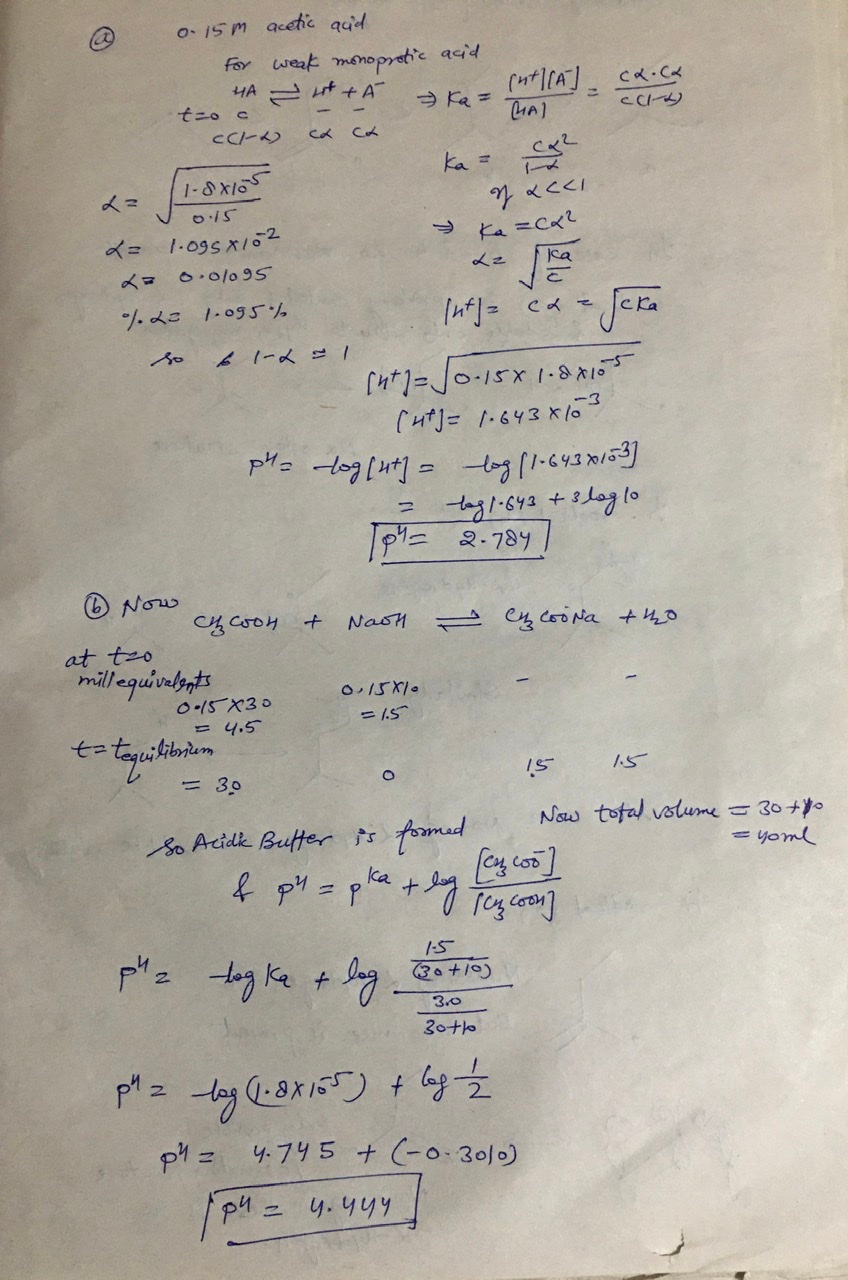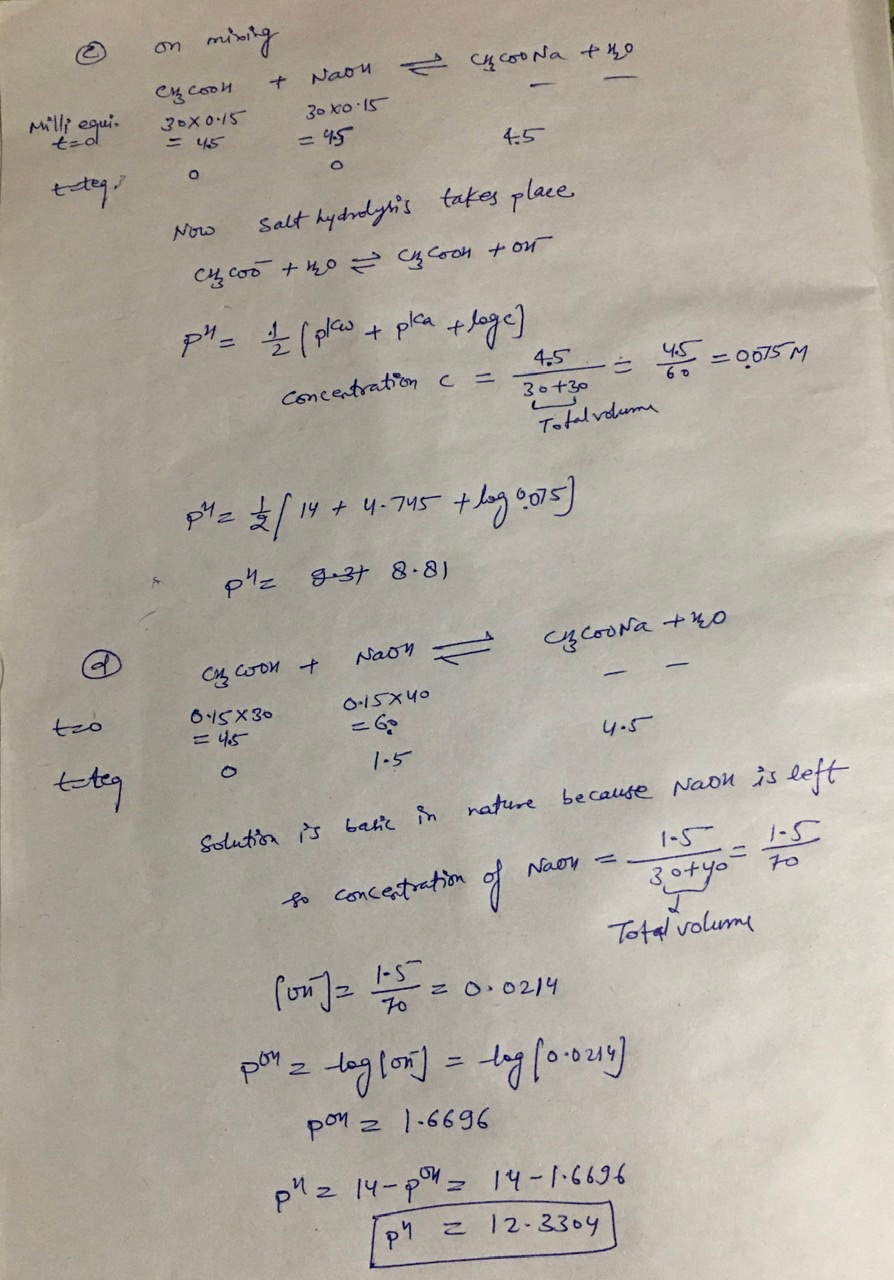#### Earn Coins

Coins can be redeemed for fabulous gifts.

Similar Homework Help Questions
• ### 8. What is the pH of a solution made by mixing 29.00 mL of 0.15 M...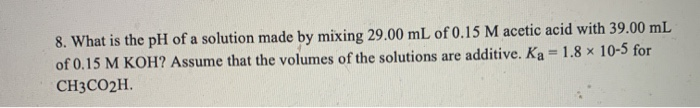8. What is the pH of a solution made by mixing 29.00 mL of 0.15 M acetic acid with 39.00 mL of 0.15 M KOH? Assume that the volumes of the solutions are additive. Ka = 1.8 x 10-5 for CH3CO2H.

• ### 7. What is the pH of a solution made by mixing 29.00 mL of 0.15 M...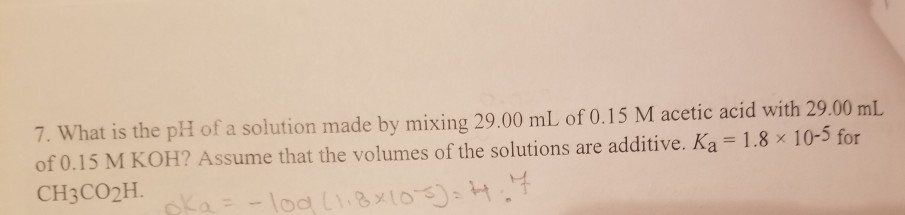7. What is the pH of a solution made by mixing 29.00 mL of 0.15 M acetic acid with 29.00 mL of 0.15 M KOH? Assume that the volumes of the solutions are additive. Ka= 1.8 x 10-5 for CH3CO2H oka-loa 8x10

• ### What is the pH of a solution prepared by mixing 30.00 mL of 0.10 MCH3CO2H with...

What is the pH of a solution prepared by mixing 30.00 mL of 0.10 MCH3CO2H with 30.00 mL of 0.040 MCH3CO2K? Assume that the volume of the solutions are additive and that Ka=1.8×10?5 for CH3CO2H.

• ### What is the pH of the solution obtained by mixing 30.00 mL of 0.250 M...

What is the pH of the solution obtained by mixing 30.00 mL of 0.250 M HCl and 30.00 mL of 0.125 M NaOH? We assume additive volumes. What is the pH of a solution that is 0.75 M in sodium acetate and 0.50 M in acetic acid? (ka for acetic acid is 1.3x10-5.) Calculate the pH of a solution prepared by mixing 15.00 ml of 0.10 M NaOH and 30.00 mL of 0.10 M benzoic acid solution. (Benzoic acid is monoprotic; its...

• ### What is the pH of a solution made by mixing 40.00 mL of 0.100 M HCl...

What is the pH of a solution made by mixing 40.00 mL of 0.100 M HCl with 35.00 mL of 0.100 M KOH? Assume that the volumes of the solutions are additive. 1.64 10.00 12.36 13.36 2.17

• ### What is the pH of the resulting solution if 30.00 mL of 0.10 M acetic acid...

What is the pH of the resulting solution if 30.00 mL of 0.10 M acetic acid is added to 30.00 mL of 0.10 M NaOH? Ka = 1.8x10-5 for CH3CO2H.

• ### Question 22 (1 point) The K, of hypochlorous acid (HCIO) is 3.0 * 10-at 25.0 "C....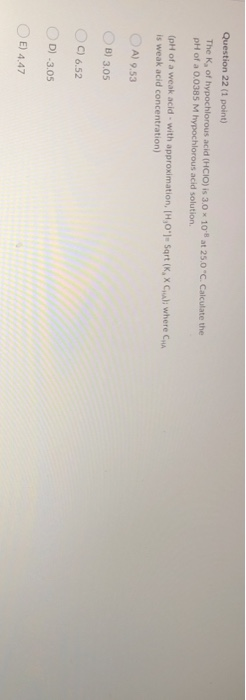Question 22 (1 point) The K, of hypochlorous acid (HCIO) is 3.0 * 10-at 25.0 "C. Calculate the pH of a 0.0385 M hypochlorous acid solution (pH of a weak acid - with approximation, [H,0") Sort (K, Cal; where CHA is weak acid concentration) A) 9.53 B) 3.05 C) 6.52 D-3.05 E) 4.47 Question 23 (1 point) A particular first-order reaction has a rate constant of 1.35 "C. What is the magnitude of kat 75.0 "CIT 105 at 25.0 (Use...

• ### What is the "What is the pH of the solution obtained by mixing 30.00 mL of 0.250 M HCl and 30.00 mL of 0.125 M NaOH?

2. What is the "What is the pH of the solution obtained by mixing 30.00 mL of 0.250 M HCl and 30.00 mL of 0.125 M NaOH? We assume additive volumes. 3. What is the pH of a solution that is 0.75 M in sodium acetate and 0.50 M in acetic acid? (K, for acetic acid is 1.8x10-5.)

• ### What is the PH of a solution made by mixing 30.00 mL of 0.10 M HCI...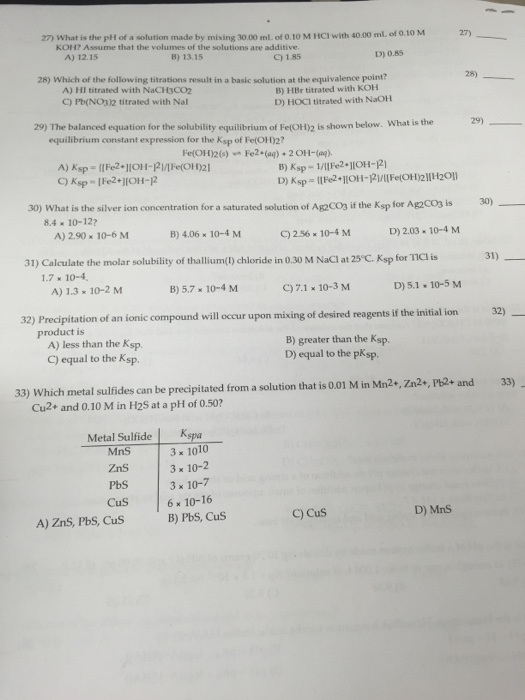What is the PH of a solution made by mixing 30.00 mL of 0.10 M HCI with 40.00 mL of 0.10 M KOH? Assume that the volumes of the solutions are additive. A) 12.15 B) 13.15 C) 1.85 D) 0.85 28) Which of the following titration result in a basic soltuion at the equilvalence point? 29) The balanced equation for the solubility equilibrium of Fe (OH)_2 is shown below. What is the equilibrium constant expression for the K_sp of (OH)_2?

• ### Consider the titration of 100.0 mL of 0.200 M acetic acid ( Ka = 1.8 x...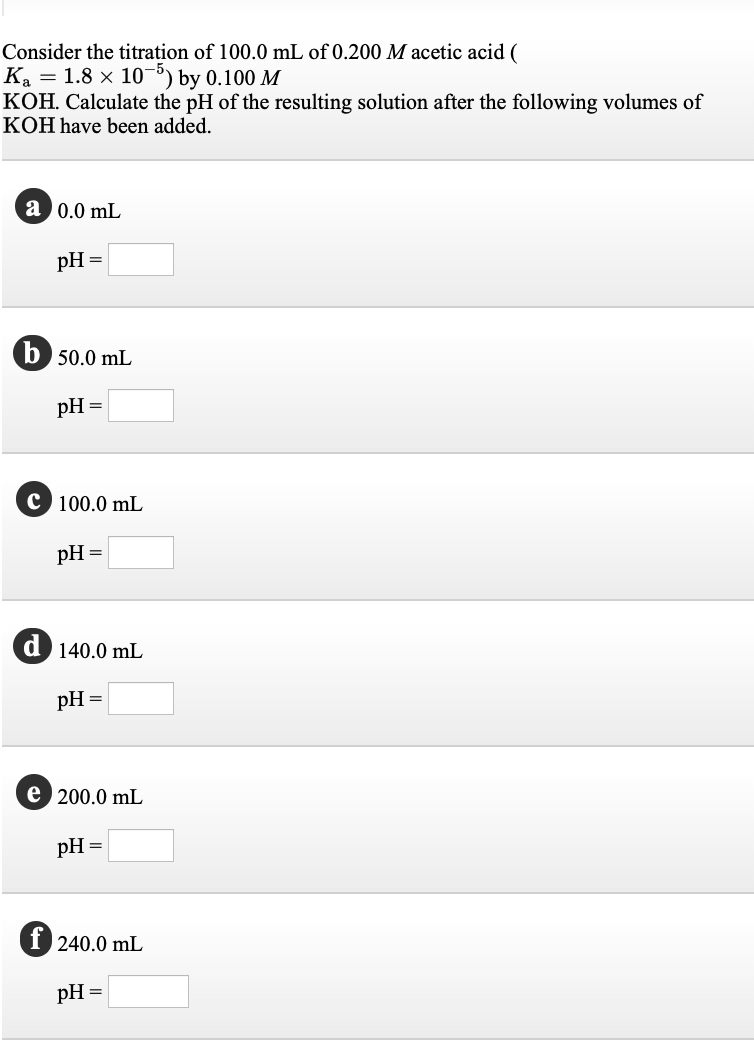Consider the titration of 100.0 mL of 0.200 M acetic acid ( Ka = 1.8 x 10-5) by 0.100 M KOH. Calculate the pH of the resulting solution after the following volumes of KOH have been added. a 0.0 mL pH= 50.0 mL pH = C 100.0 mL pH = 140.0 mL pH= C 200.0 mL pH = f 240.0 mL pH=